# Texas Go Math Grade 3 Lesson 14.7 Answer Key Find Unknown Numbers

Refer to our Texas Go Math Grade 3 Answer Key Pdf to score good marks in the exams. Test yourself by practicing the problems from Texas Go Math Grade 3 Lesson 14.7 Answer Key Find Unknown Numbers.

## Texas Go Math Grade 3 Lesson 14.7 Answer Key Find Unknown Numbers

Unlock the Problem

Tanisha plans to invite 24 people to a picnic. The invitations come in packs of 8. How many packs of invitations does Tanisha need to buy?

An equation is a number sentence that uses the equal sign to show that two amounts are equal.

A symbol or letter can stand for an unknown factor. You can write the equation, n × 8 = 24, to find how many packs of invitations Tanisha needs. Find the number, n, that makes the equation true.

How many people is Tan isha inviting? _________________

How many invitations are in 1 pack? _________________

One Way Use an array.

Show an array with 24 tiles with 8 tiles in each row by completing the drawing.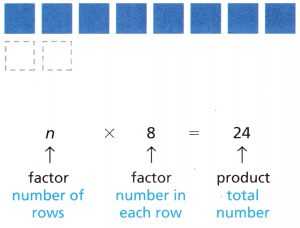Count how many rows of 8 tiles there are.
Think: What number times 8 equals 24?
There are _______ rows of 8 tiles. The unknown factor is _______. n = _________
_______ × 8 = 24 Check.
_______ = 24 ✓ The equation is true.
So, Tanisha needs _________ packs of invitations.
There are 3 rows of 8 tiles. The unknown factor is 3. n = 3
3 × 8 = 24 Check.
n = 24 ✓ The equation is true.
So, Tanisha needs 3 packs of invitations.

Another Way Use a multiplication table.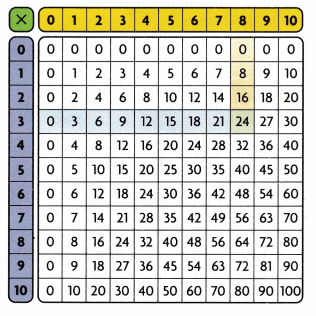24 ÷ 8 =Think:× 8 = 24
Find the factor, 8, in the top row.
Look down to find the product, 24.
Look left across the row from 24.
The unknown factor is _______.= _______
_______ × 8 = 24 Check.
_______ = 24 ✓ The equation is true.
Explanation:
24 ÷ 8 = 3
Think: 3 × 8 = 24
The unknown factor is 3.
n = 3
3 × 8 = 24 Check.
n = 24 ✓ The equation is true.

Share and Show

Question 1.
What is the unknown factor shown by this array?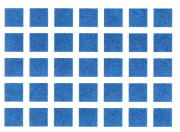35 ÷= 5= _________
Explanation:
35 ÷ 7 = 5= 7 is the unknown factor shown by this array

Find the unknown number.

Question 2.
d × 3 = 27
d = __________
Explanation:
27 ÷ 3 = 9
so d = 9

Go Math Grade 3 Find An Unknown Number Question 3.
30 ÷= 6= __________
Explanation:
5 x 6 = 30= 5

Question 4.
c = 4 × 5
c = _________
c = 20
Explanation:
The  product of 4 x 5 = 20

Question 5.× 2 = 14= _________= 7
Explanation:
14 ÷  2 = 7

Question 6.
36 ÷ 9 = b
b = __________
b = 9
Explanation:
9 x 4 = 36

Question 7.
8 × e = 64
e = __________
Explanation:
8 x 8 = 64
e = 8

Question 8.
7 ×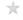= 42= _________= 6
Explanation:
The product of 7 x 6 = 42

Question 9.
8 × 9 = z
z = _________
z = 72
Explanation:
The product of 8 and 9 is 72

Question 10.÷ 6 = 3= __________= 18
Explanation:
The product of 3 and 6 is 18

Question 11.
36 = p × 6
p = ________
p = 6
Explanation:
36 ÷ 6 = 6

Question 12.
9 × 7 = m
m = ________
m = 63
Explanation:
The product of 9 and 7 = 63

Question 13.
40 ÷= 4= __________= 10
Explanation:
The product of 4 and 10 is 40

Problem Solving

H.O.T. Algebra Find the unknown number.

Question 14.
3 × 6 = k × 9
k = ________
Explanation:
3 × 6 = k × 9
18 = k × 9
k = 18 ÷  9
k = 3 is the unknown number

Question 15.
4 × y = 2 × 6
y = __________
Explanation:
4 × y = 2 × 6
4 × y = 12
y = 12 ÷  4
y = 3 is the unknown number

30 ÷ g = 36 – 26
g = __________
30 ÷ g = 36 – 26
30 ÷ g =10
g = 30 ÷  10
g = 3 is the unknown number

Question 17.
2 × 4 =÷ 3= __________
Explanation:
2 × 4 =÷ 3
8  =÷ 3= 8 x 3= 24 is the unknown number

Question 18.
9 × d = 70 + 2
d = __________
Explanation:
9 × d = 70 + 2
9 × d = 72
d = 72 ÷  9
d = 8 is the unknown number

Question 19.
8 × h = 60 – 4
h = _______
Explanation:
8 × h = 60 – 4
8 × h = 56
h = 56 ÷ 8
h = 7 is the unknown number

Problem Solving

Use the table for 20-23.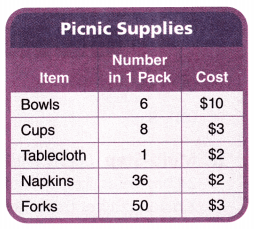Question 20.
Tanisha needs 40 cups for the picnic. How many packs of cups should she buy?
Explanation:
5 x 8 = 40
1 pack has 8 cups
she should buy 5 pack of cups

Question 21.
H.O.T. Write Math What if Tanisha needs 40 bowls for the picnic? Explain how to write an equation with a letter for an unknown factor to find the number of packs she should buy. Then find the unknown factor.Tanisha needs 40 bowls
6 x n = 42
n = 42 ÷ 6
n = 8
so , she should buy 8 packs

Question 22.
Multi-Step Ms. Hill buys 3 tablecloths and 2 packs of napkins. How much money does she spend?
1 table cloth = $2 3 x 2 = 6 pack of 36 napkins is 2$
2 x 2$=$ 4
6 + 4 = 10$she spend on 3 tablecloths and 2 packs of napkins. Question 23. H.O.T. What if Randy needs an equal number of bowls and cups for his picnic? How many packs of each will he need to buy? Answer: 48 = 48 Explanation 1 pack of bowls = 6 1 pack of cups = 8 she needs to buy 8 packs of bowls and 6 packs of cups 6 x 8 = 8 x 6 48 = 48 Daily Assessment Task Fill in the bubble for the correct answer choice. You can use arrays or equations to represent and solve. Question 24. What is the unknown product? a = 5 × 9 (A) 30 (B) 45 (C) 14 (D) 40 Answer: B Explanation: The product of 5 x 9 = 45 Question 25. Representations The first row of an array is shown below. If the entire array has 40 tiles, how many rows are in the array?(A) 35 (B) 8 (C) 5 (D) 12 Answer: B Explanation: Number of columns are 5 40 ÷ 5 = 8 Question 26. Multi-Step Lenny and Kristen each made a batch of 24 brownies. Lenny cut his brownies into 3 equal rows. Kristen cut her brownies into 4 equal rows. How many more brownies are in each of Lenny’s rows than are in Kristen’s rows? (A) 6 (B) 2 (C) 1 (D) 8 Answer: 2 Explanation: 2 more brownies are in each of Lenny’s rows than are in Kristen’s rows 3 x 8 = 24 4 x 6 = 24 Texas Test Prep Question 27. What is the unknown factor? m × 6 = 48 (A) 8 (B) 6 (C) 7 (D) 5 Answer: A Explanation: m = 48 ÷ 6 m = 8 ### Texas Go Math Grade 3 Lesson 14.7 Homework and Practice Answer Key Find the unknown number. Question 1. b × 4 = 32 b = __________ Answer: 8 b = 8 Explanation: 32 ÷ 4 = 8 is the unknown number Question 2. m ÷ 3 = 7 m = __________ Answer: 21 Explanation: m = 21 The product of 7 and 3 is 21 Question 3.× 7 = 49= __________ Answer: 7= 7 Explanation: 49 ÷ 7 = 7 is the unknown number Question 4. 20 ÷ 5 = y × 2 y = __________ Answer: 2 y = 2 Explanation: 20 ÷ 5 = y × 2 4 = y x 2 y = 4 ÷2 = 2 is the unknown number Question 5. h × 6 = 9 × 4 h = __________ Answer: 6 Explanation: h x 6 = 36 h = 36 ÷ 6 h = 6 is the unknown number Question 6. 8 ×= 40 + 8= __________ Answer: 6 8 ×= 40 + 8 8 ×6 = 48= 6 is the unknown number Problem Solving Use the table for 7-10.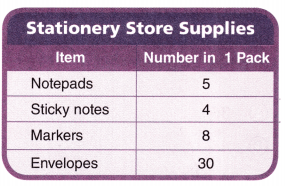Question 7. Miss LaBlanc needs 32 markers for her art class. How many packs should she buy? Answer: 4 Explanation: Miss LaBlanc needs 32 markers for her art class. 4 packs she should buy. 1 pack of markers has 8 8 x 4 = 32 Question 8. Brian spent$18 on sticky notes and envelopes. Each pack of sticky notes costs $3. A pack of envelopes costs$6 If he buys an equal number of packs of each, how many packs of each did Brian buy?
Brian spent $18 on sticky notes and envelopes. Each pack of sticky notes costs$3.
A pack of envelopes costs $6 If he buys an equal number of packs of each, sticky notes = 6 and envelopes = 3 packs of each should Brian buy. Question 9. Joshua needs an equal number of notepads and sticky notes. How many packs of each item does Joshua need to buy? Answer: 5 x 4 = 20 4 x 5 = 20 20 = 20 Explanation: Joshua needs an equal number of notepads and sticky notes. 4 packs of notepads and 5 packs of sticky notes for each item Joshua needs to buy. Go Math Grade 3 Pdf Download Lesson 14.7 Homework Answer Key Question 10. Mr. Garcia needs 28 notepads for his class. Explain how to write an equation with a letter for the unknown factor to find how many packs he should buy. Then find the unknown factor. Answer: 1 pack of note pads = 5 We cannot find for 28 so, taken nearby 10 that is 30 n x 6 = 30 n = 30 ÷ 6 n = 5 Lesson Check Fill in the bubble completely to show your answer. Question 11. Fiona does 30 sit-ups in sets of 5 sit-ups each. How many sets of sit-ups does Fiona do? (A) 35 (B) 5 (C) 25 (D) 6 Answer: D Explanation: Fiona does 30 sit-ups in sets of 5 sit-ups each. 6 sets of sit-ups done by Fiona 5 x 6 = 30 Question 12. Kalon does 48 jumping jacks in sets of 6 jumping jacks each. How many sets of jumping jacks does Kalon do? (A) 6 (B) 8 (C) 46 (D) 40 Answer: B Explanation: Kalon does 48 jumping jacks in sets of 6 jumping jacks each. 8 sets of jumping jacks done by Kalon. Question 13. Rich draws this first row of an array. If the entire array has 36 circles, how many rows are there in the array?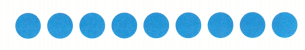(A) 36 (B) 45 (C) 4 (D) 6 Answer: C Explanation: Rich draws this first row of an array. If the entire array has 36 circles, 4 rows are there in the array 9 x 4 = 36 Question 14. Mrs. Spencer arranges one row of 7 chairs in her classroom. If she arranges 28 chairs in equal rows, how many rows of chairs are there? (A) 4 (B) 7 (C) 35 (D) 28 Answer: A Explanation: Mrs. Spencer arranges one row of 7 chairs in her classroom. If she arranges 28 chairs in equal rows, 4 rows of chairs are there 7 x 4 = 28 Question 15. Multi-Step Coz and Amelia each make a tile design with 36 tiles. Coz puts his in rows of 4. Amelia puts hers in rows of 6. How many more tiles are in each of Coz’s rows than Amelia’s? (A) 6 (B) 9 (C) 3 (D) 2 Answer: C Explanation: Coz and Amelia each make a tile design with 36 tiles. Coz puts his in rows of 4. Amelia puts hers in rows of 6. 3 more tiles are in each of Coz’s rows than Amelia’s 4 x 9 = 36 6 x 6 = 36 9 – 6 = 3 Question 16. Multi-Step Denny buys 3 boxes of cereal for$4 each and 5 boxes of oatmeal for $3 each. How much money does Denny spend in all? (A)$15
(B) $7 (C)$27
(D) $8 Answer: C Explanation: Denny buys 3 boxes of cereal for$4 each and 5 boxes of oatmeal for $3 each. 4 x 3 = 12 5 x 3 = 15 12 + 15 = 27$